### Prioritizing Using Exponential Decay

I had an officer ask the other day if I could identify the top 10 subjects in our records management system that were involved in mental health occurrences. On the surface this is a straightforward request: identify the people with the most activity but it gets trickier when you factor in the passage of time. For example, is someone who had 3 incidents over 6 months ago more of a concern than someone who had 2 incidents in the last 8 weeks? Clearly recency should factor into how a person is prioritized but history should also weigh in. To address questions like this we can use an exponential decay equation in Excel to preferentially weight recent incidents while still giving some weight to the past. In this post I will outline how to apply the method.

The first step is to obtain some data. Depending on the nature of the incident I will set an arbitary historical cut-off; in this example we’ll set it a year. This then means that we need to extract every mental health occurrence over the last year along with the date of the occurrence and a unique person identifier.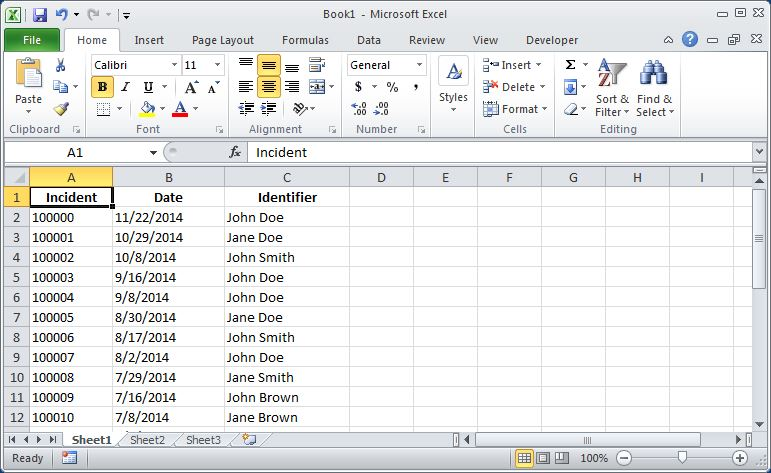For each occurrence we then calculate the age of the incident in days by subtracting the date of the occurrence from today. This is done using Excel’s NOW() function to get the current date and the INT() function to trim off the time since we are working strictly with days. the following screen shot shows the formula in the function bar.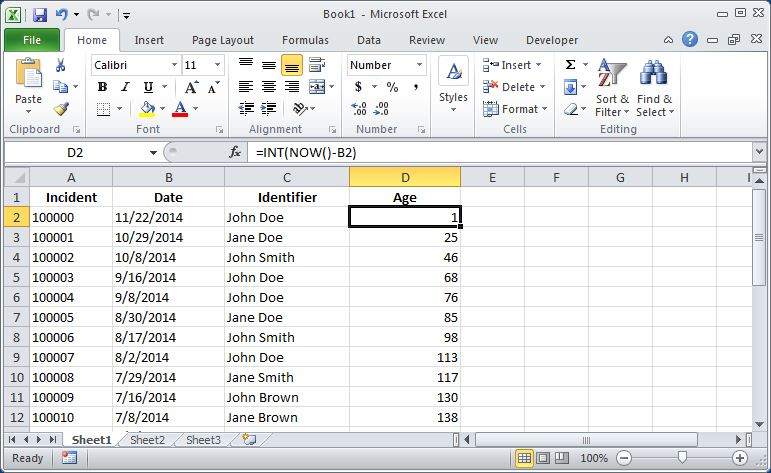Next we want to create a column for the exponential decay formula. Most people are familiar with the idea of exponential decay, it is a mathematical formula where a quantity decreases at a rate proportional to its current value. In practice that means that the value drops off quickly and first and then more slowly as the value shrinks. The chart below shows a graph of exponential decay for a number of different constants. As can be seen in the chart we can tune how fast the values drop off by adjusting the constant.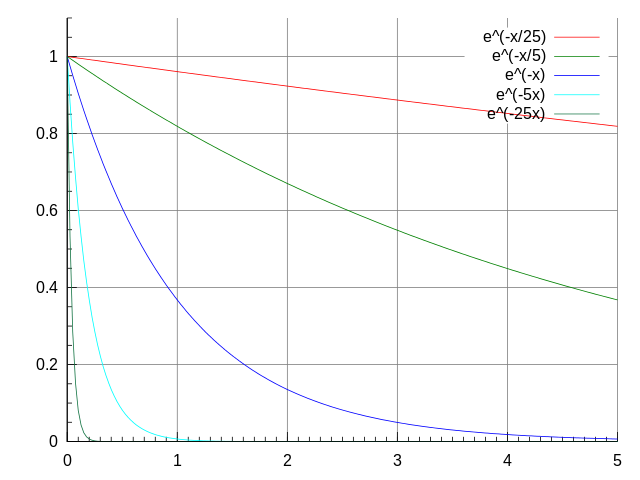In Excel, the formula for exponential decay is =EXP(-constant*time) where we get to specify the constant and time, in our case, is the age of the incident. Since we’re dealing with days and we only have a year of data we can decide how much we want past incidents to influence a person’s priority. For example, if we say that a mental health incident that happened today is worth 1 point, how much is an incident that happened 6 months ago worth? If we say that it’s work 0.5 points then we can calculate our constant as: -1*LN(0.5)/180. Where LN() is Excel’s natural logarithm function, 0.5 is point value we want to calculate and 180 is the (approximate) number of days in 6 months. This calculation results in a value of 0.003851 which we can use as our constant.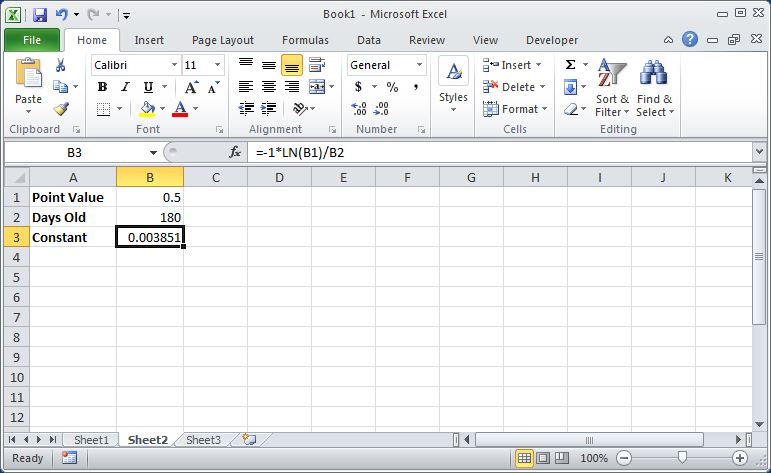Back in our data worksheet we can finally create our new column that calculates the decay score for each incident based on its age. We use the exponential decay formula =EXP(-1*0.003851*age) to calculate the decay score for each incident as in the following screen shot. Note that the decay value will always be less than 0 but never reach 0.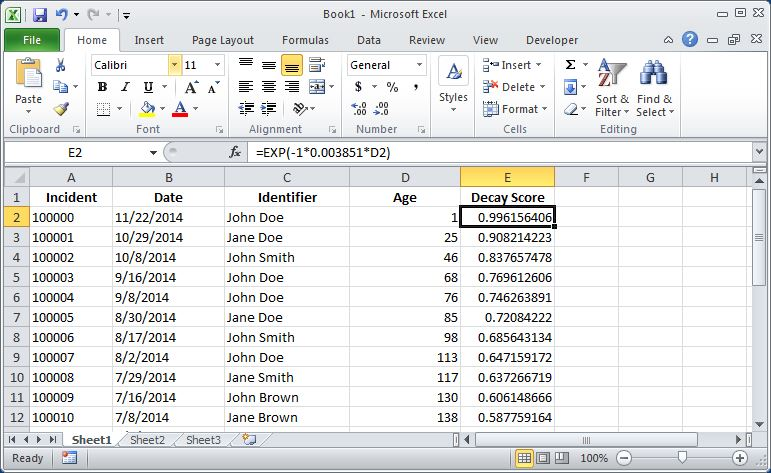Now that we have a decay score for each occurrence we need to create a pivot table of all the data. Highlight everything and select ‘PivotTable’ from the ‘Insert’ tab. This will open a modal window and you can just hit the ‘OK’ button to create your pivot worksheet.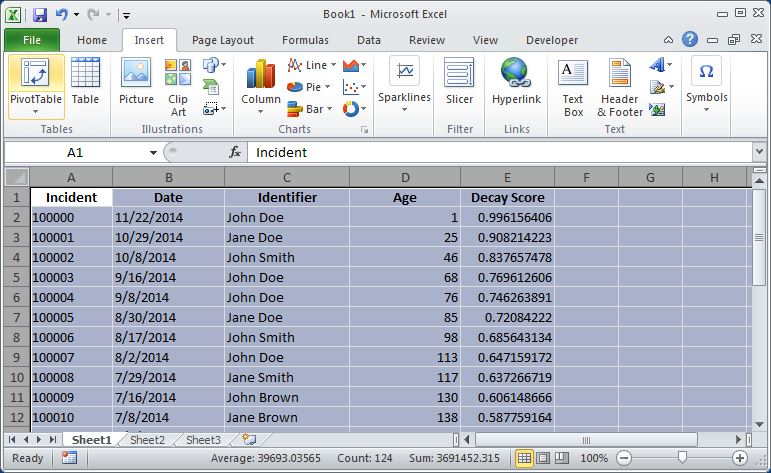On the pivot table worksheet drag the Identifier field to the Row Labels box and the Decay Score field to the Values box. Click on ‘Count of Decay Score’ in the Values box and select the ‘Value Field Settings’ option at the bottom of the pop-up window. Change the selected option from Count to Sum and click ‘OK’.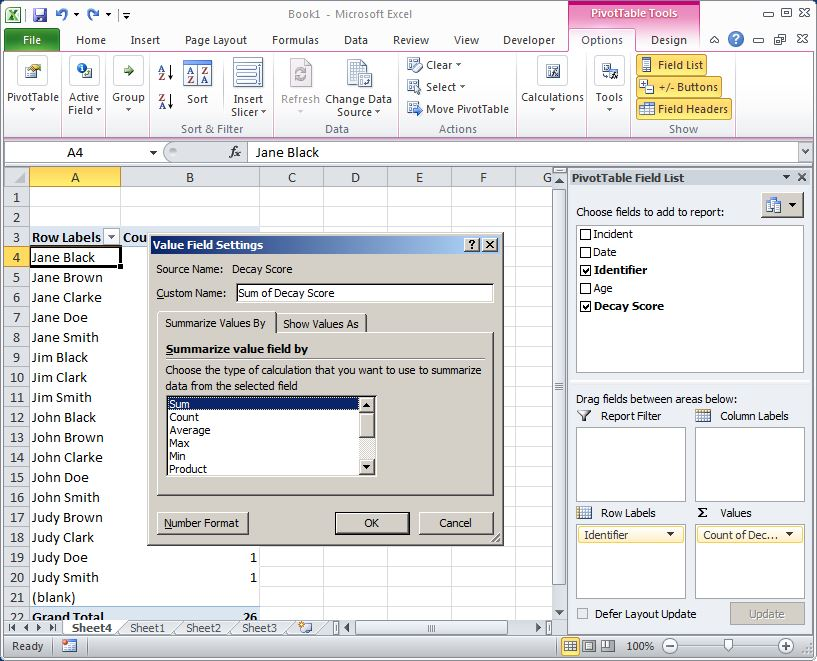Almost done. Back in your pivot table right-click the Sum of Decay Score column and sort ‘Largest to Smallest’. This will sort the persons by their aggregated decay scores and the people at the top are the highest priority individuals.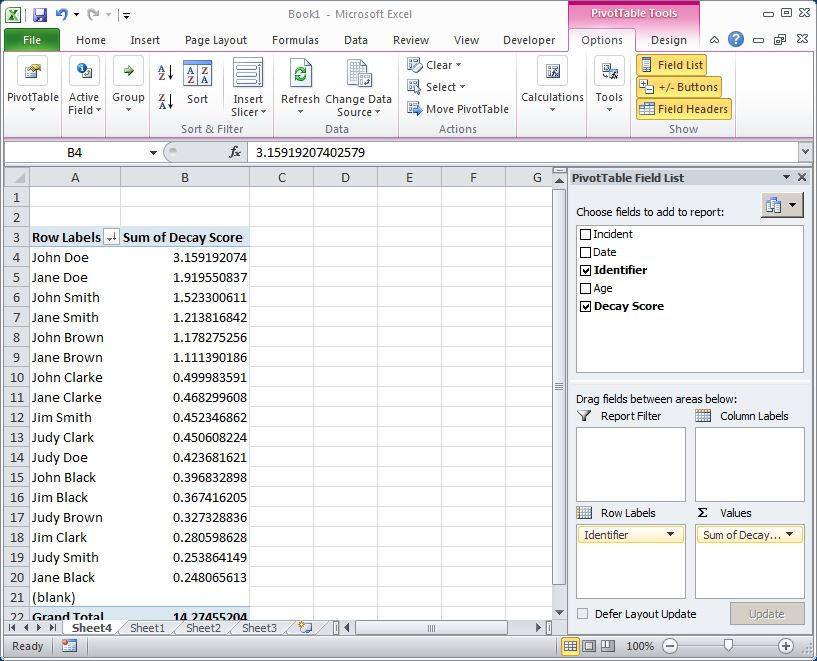This method works because the exponential function embodies the principles we were originally trying to capture: it gives more weight (a higher score) to recent incidents but it also gives some score to historic incidents as well. For every occurrence a person is involved with they have the potential to add up to 1 point to their total score (if the incident happened just today) but as the incidents age they influence the total score less. In our example above the person with the 3 incidents over six months ago has, at most, a score of 1.5 (since 0.5 is the 6 month mark) while our 2 incidents in the last 8 weeks person is likely to have a score a little less than 2. So this system weights these people the same by balancing the influence of recent and history. The best part is that we can tune how much weight we give to the two factors by adjusting the exponential decay constant. If we decide that an incident 6 months is only worth 0.1 point rather than 0.5, which greatly privileges recency over history, we can adjust the constant and rescore everyone. On the other hand, if we wanted to make a 6 month incident worth 0.9 points, we could recalculate the scores and privilege history over recency.

I like this method of prioritizing because it’s simple to implement in Excel (no plug-ins!), it’s logically justifiable and it allows the impact of recency and history to vary according to the expertise of the analyst. I hope you find it useful and good luck prioritizing!